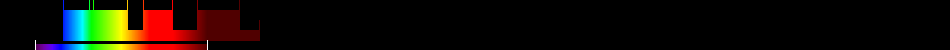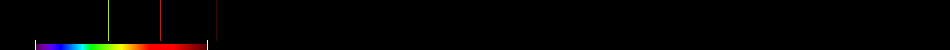# Information for Sensor and Index

## Formula

General Formula $\left(1.5\frac{2.5\left({\mathrm{800nm}}-{\mathrm{670nm}}\right)-1.3\left({\mathrm{800nm}}-{\mathrm{550nm}}\right)}{\sqrt{{\left(2{\mathrm{800nm}}+1\right)}^{2}-\left(6{\mathrm{800nm}}-5\sqrt{{\mathrm{670nm}}}\right)-0.5}}\right)$ $\left(1.5\frac{2.5\left({\mathrm{nahe_Infrarot}}-{\mathrm{Rot}}\right)-1.3\left({\mathrm{nahe_Infrarot}}-{\mathrm{GrÃ¼n}}\right)}{\sqrt{{\left(2{\mathrm{nahe_Infrarot}}+1\right)}^{2}-\left(6{\mathrm{nahe_Infrarot}}-5\sqrt{{\mathrm{Rot}}}\right)-0.5}}\right)$ Automatic

## Sensor

Name IKONOS-2 5 445-900 0.82-3.2 98.1 GeoEye 1999-09-24 original IKONOS-1 was lost during launch at 1999-04-27 yes

## Index

Name Modified Chlorophyll Absorption in Reflectance Index 2 MCARI2 $\left(1.5\frac{2.5\left({\mathrm{800nm}}-{\mathrm{670nm}}\right)-1.3\left({\mathrm{800nm}}-{\mathrm{550nm}}\right)}{\sqrt{{\left(2{\mathrm{800nm}}+1\right)}^{2}-\left(6{\mathrm{800nm}}-5\sqrt{{\mathrm{670nm}}}\right)-0.5}}\right)$ 550,670,800 Original formula

## References